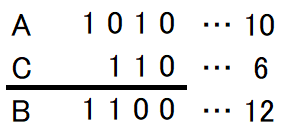﻿ ABC213 A - Bitwise Exclusive Or - Atcoder

# Home

Score : $100$ points

### Problem Statement

You are given integers $A$ and $B$ between $0$ and $255$ (inclusive). Find a non-negative integer $C$ such that $A \text{ xor }C=B$.

It can be proved that there uniquely exists such $C$, and it will be between $0$ and $255$ (inclusive).

What is bitwise $\mathrm{XOR}$?

The bitwise $\mathrm{XOR}$ of integers $A$ and $B$, $A\ \mathrm{XOR}\ B$, is defined as follows:

• When $A\ \mathrm{XOR}\ B$ is written in base two, the digit in the $2^k$'s place ($k \geq 0$) is $1$ if exactly one of $A$ and $B$ is $1$, and $0$ otherwise.
For example, we have $3\ \mathrm{XOR}\ 5 = 6$ (in base two: $011\ \mathrm{XOR}\ 101 = 110$).

### Constraints

• $0\leq A,B \leq 255$
• All values in input are integers.

### Input

Input is given from Standard Input in the following format:

$A$ $B$


### Sample Input 1

3 6


### Sample Output 1

5


When written in binary, $3$ will be $11$, and $5$ will be $101$. Thus, their $\text{xor}$ will be $110$ in binary, or $6$ in decimal.

In short, $3 \text{ xor } 5 = 6$, so the answer is $5$.

### Sample Input 2

10 12


### Sample Output 2

6